Courses

# Operational Amplifiers - 2 Notes | EduRev

## Electronics and Communication Engineering (ECE) : Operational Amplifiers - 2 Notes | EduRev

The document Operational Amplifiers - 2 Notes | EduRev is a part of the Electronics and Communication Engineering (ECE) Course Analog Circuits.
All you need of Electronics and Communication Engineering (ECE) at this link: Electronics and Communication Engineering (ECE)

Schmitt Trigger

The Schmitt trigger or Bistable multivibrator uses positive feedback with loop gain greater than unity to produce a bistable characteristics as shown below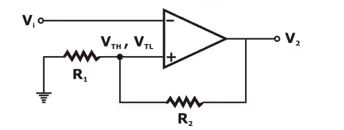Inverting Schmitt Trigger

Here,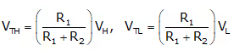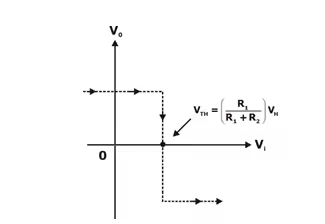Voltage Transfer Characteristics as input voltage increases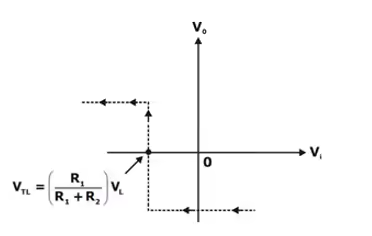Voltage Transfer Characteristics as input voltage decreases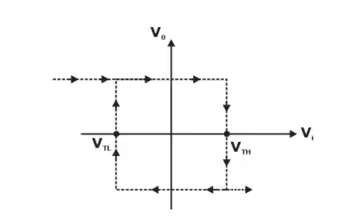Net Voltage Transfer Characteristics showing Hysteresis EffectHere,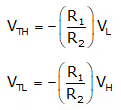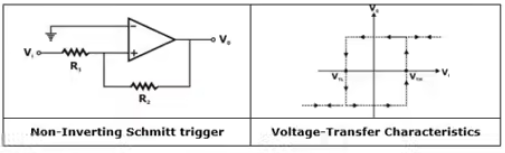Precision Rectifier

Below figure shows a Precision rectifier and its input and output waveform.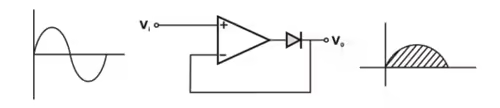For positive value of Vi, diode will be ON. and for negative value of Vi, diode will be OFF as shown below.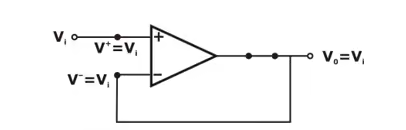Equivalent Circuit of Precision Rectifier for Positive value of Vi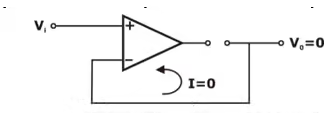Equivalent Circuit of Precision Rectifier foe Negative value of Vi

From the above circuit, we observe that
For Vi > 0, diode will be OFF, so V0 = 0
For Vi < 0, diode will be ON, so V0 = Vi
Thus, we obtain the voltage transfer characteristics for the precision rectifier as shown below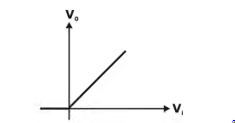Voltage Transfer Characteristics for Precision Rectifier

Instrumentation Amplifier

It consists of two non-inverting amplifiers and one difference amplifier.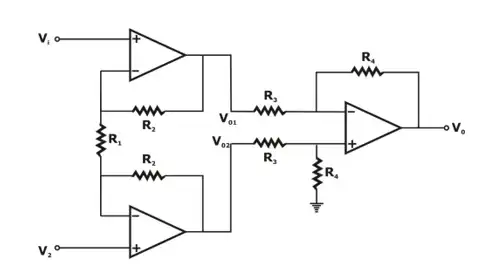Instumentation Amplifier

The output voltages of the two non-inverting amplifiers are given by: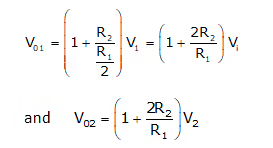Thus, we obtain the net output of the instrumentation amplifier as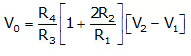Astable Multivibrator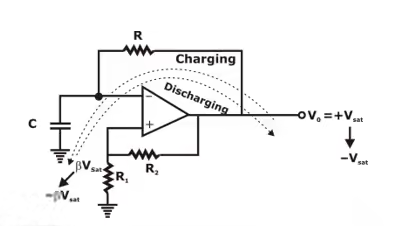Astable Multivibrator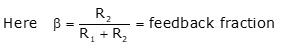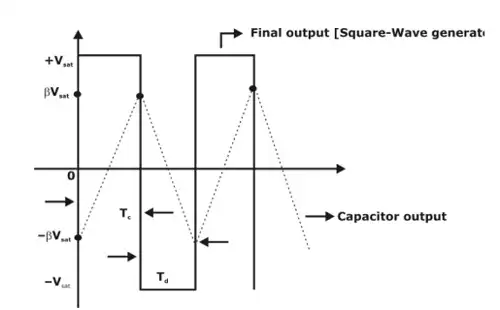Waveform

Here,
TC = charging time
Td = discharging time
V0 = Vfinal + [Vinitial – Vfinal]e–t/RC
Here,
Vinitial = βVsat
Vfinal = –Vsat
V0 = –βVsat
∴ –βVsat = –Vsat + [βVsat + Vsat]e–Td/RC
(1 – β) Vsat = (1 + β) Vsat .e–Td/RC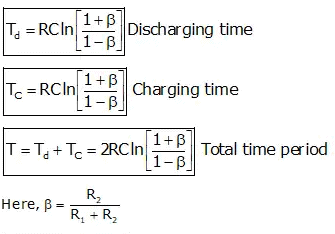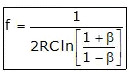Frequency of square wave generator

Bistable Multivibrator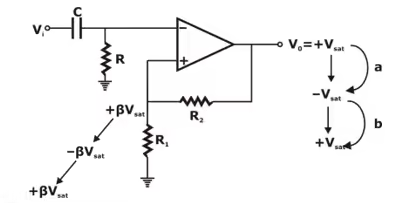Bistable Multivibrator

Here, a to b changes only after triggering and before triggering, it will be
constant = +Vsat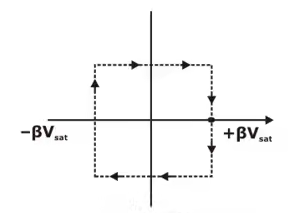Monostable Multivibrator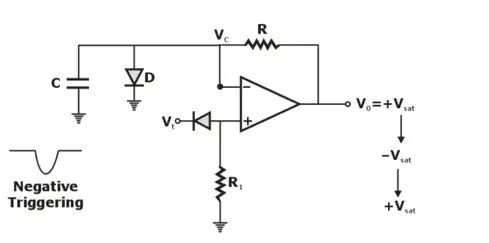Monostable Multivibrator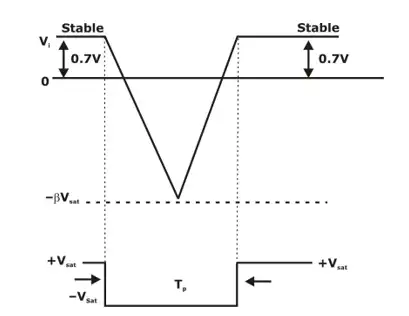Waveform

Time Period of Monostable Multivibrator:
TP = 0.693RC

Offer running on EduRev: Apply code STAYHOME200 to get INR 200 off on our premium plan EduRev Infinity!

## Analog Circuits

1 videos|16 docs|43 tests

,

,

,

,

,

,

,

,

,

,

,

,

,

,

,

,

,

,

,

,

,

;